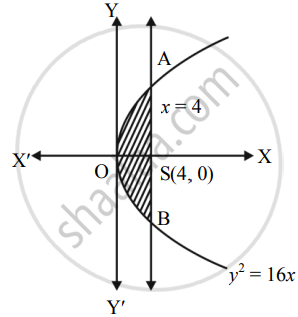# Find the area of the region bounded by the parabola y2 = 16x and the line x = 4 - Mathematics and Statistics

Sum

Find the area of the region bounded by the parabola y2 = 16x and the line x = 4

#### Solution

Given equation of the curve is y2 = 16x

y2 =

∴ y = +-  4sqrt(x)

∴ y = 4 sqrt(x)    ......[∵ In first quadrant, y > 0]Required area = Area of the region OBSAO

= 2   .......(Area of the region OSAO)

= 2int_0^4 y  "d"x

= 2 int_0^4 4sqrt(x)  "d"x

= 8[(x^(3/2))/(3/2)]_0^4

= 16/3[(4)^(3/2) - 0]

= 16/3(8)

= 128/3 sq.units

Concept: Area Bounded by the Curve, Axis and Line
Is there an error in this question or solution?